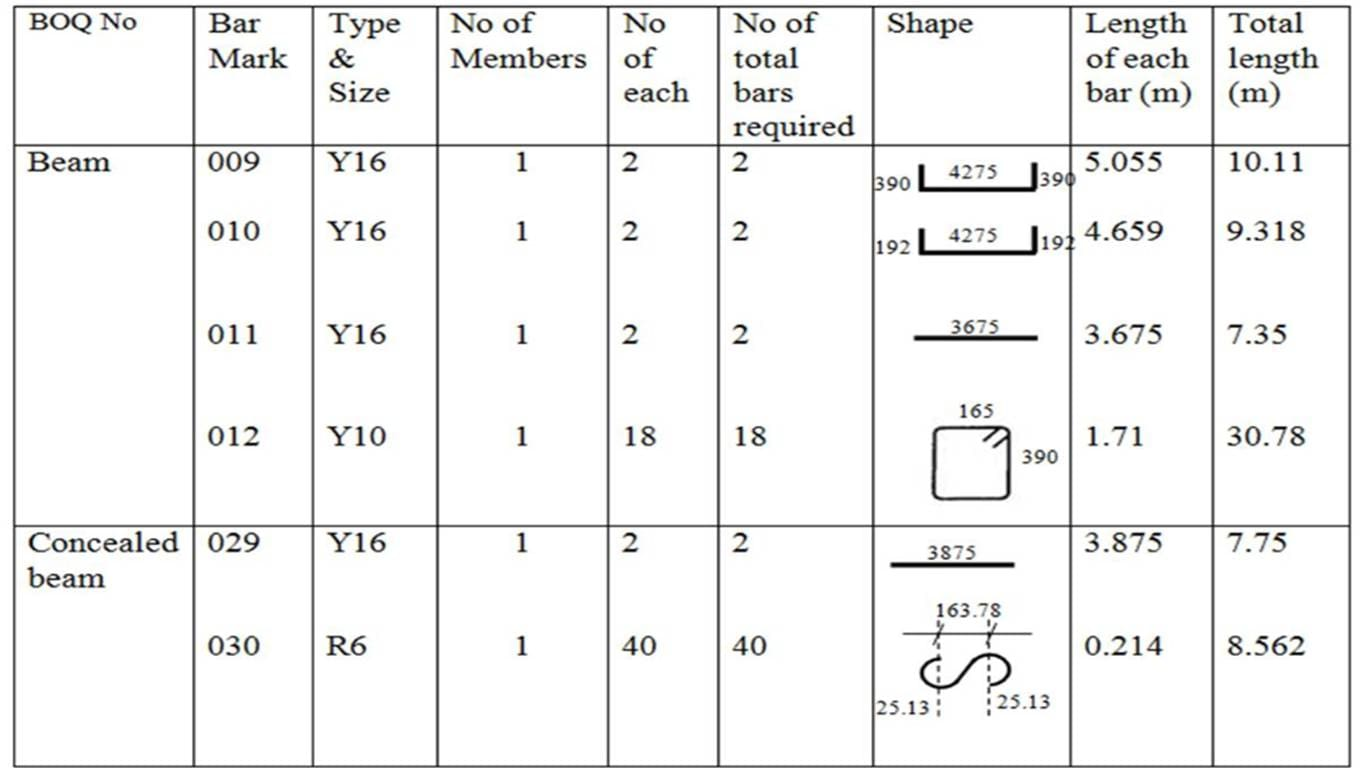# How to Calculate (Formula) for Unit Weight of Reinforcement Steel Bar?

## Why Weight of Steel is required while Construction?

The quantity of Steel is usually derived from the drawings using Bar Bending Schedule (Sample Bar Bending Chart shown below). The quantity of Steel arrived used bar bending schedule is usually in length of each dia of bar required. But Steel is always procured or brought in weight (kgs or Tonnes).To convert the required length of Steel bar into weight, One needs to understand how to calculate the unit weight of a reinforcement steel bar. With this formula it becomes easy for engineers and home builders to calculate the steel requirement for the construction.

## how to derive The Formula For Weight of Steel bar?

Many reading this blog might know that the unit weight of a steel bar is calculated by a forumla D^2/162 (Note: D unit is in MM) but do you know how its derived?

Density of Steel (in Kgs/Cu.m) = Weight of Steel (in Kgs)/Volume of Steel (in Cu.m)

So,

Weight of Bar (in Kgs) = Volume of Steel Bar (in Cu.m) X Density of Steel Bar (in Kgs/Cu.m)

Data:

Volume of Steel Bar (Cu.m) = Area of Steel Bar (sq.mtr) X Length of Steel Bar (mtr)

Since We are trying to calculate the unit weight of Steel Bar, the length of Steel Bar in the calculation would be 1 mtr or 1000 mm.

Area of Steel Bar = (3.14 X D^2)/4

Density of Steel Bar = 7850 Kgs/Cu.m = 7850/(1000X1000X1000) Cubic mm

So considering these data in the Weight formula we get

Unit Weight of Steel Bar (in Kgs) = (3.14 X D^2)/4 X 1000 X 7850 / (1000 X 1000 X 1000)

= D^2 X (0.006162)

To simplify, we can reciprocate the decimal figure here i.e., (1/0.006162) and get a figure of 162.28

Unit weight of Steel Bar (in Kgs) = (D^2)/162.28 ~ (D^2)/162

Where D is the Diameter of the Steel Bar in mm.

### Example for Calculating the Unit weight of Steel Bar?

Q: Calculate the weight of 16 mm Steel Bar of Length 12 mtrs

Ans : Since We know that the unit weight of steel can be derived from the Formula i.e., (D^2)/162

Unit weight of 16mm Steel bar (kgs/mtr) = (D^2)/162 = (16 X 16)/162 = 1.580 kgs/mtr

Since we want to calculate the weight of bar of length 12 mtrs, we multiply the unit weight with the length.

i.e., Weight of 12 mtrs length 16mm dia Steel Bar = 1.58 Kgs/ mtr X 12 mtr = 18.960 Kgs

* Always remember the the weight of Steel Bar should be approximated to 3 decimals as per IS Code.

## How to Measure/Check the Weight of Steel Received at Site?

Since it is hard to weight the bars at site, Trucks/Trailers carrying steel are weighed (at Weigh Bridges) both fully loaded with Steel (Gross weight) and empty truck (Tare weight).

Steel Weight can be calculated by the following forumla

Reinforcement Steel received at Site (in Kgs) = Gross Weight – Tare Weight

Diesel allowance (30-40 Kgs) is diesel consumed between obtaining the two weights i.e., Gross weight and Tare weight. This Diesel allownce needs to be deducted incase the weigh bridge is far away from the Site.